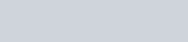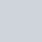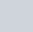From the letters of the word ”MOBILE”,
Question:

From the letters of the word ”MOBILE”,  a letter is selected. The probability that the letter is a vowel, is

(a) $\frac{1}{3}$

(b) $\frac{3}{7}$

(c) $\frac{1}{6}$

(d) $\frac{1}{2}$

Solution:

GIVEN: A letter is selected from the word “MOBILE”

TO FIND: Probability that the letter chosen is a vowel

Total letter in the word “MOBILE” is 6

Vowels in the word “MOBILE” are ‘O’.’I’,’E’

Hence total number of favorable outcome is 3 i.e. ‘O’.’I’,’E’

We know that PROBABILITY =Hence probability that the letter chosen is a vowelHence the correct option is